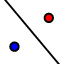# Exploring Line Reflections

## DIRECTIONS:

1) Position Wily Coyote (using points A and B) anywhere you'd like within the coordinate plane. 2) Select the REFLECT ABOUT LINEtool. Then drag a rectangle around points A, B, and Wily's picture. This should cause these 3 items to become highlighted. Select the dashed line. 3) The images of these items should now appear. Please answer the questions that appear below the applet.

## 1.

Move the ORANGE POINTS so that the dashed line lies right on top of the yAxis. What do you have as Wily's corner points ( ?, ??). Write them down below. What are the coordinates of the images of these points? Please record these below too. Do you notice anything interesting? Explain.

## 2.

According to your observations from (1), what would the image of any point (x, y) after being reflected about the yAxis? (Write your response in terms of x and y).

## 3.

Move the ORANGE POINTS so that the dashed line lies right on top of the xAxis. What do you have as Wily's corner points ( ?, ??). Write them down below. What are the coordinates of the images of these points? Please record these below too. Do you notice anything interesting? Explain.

## 4.

According to your observations from (1), what would the image of any point (x, y) after being reflected about the xAxis? (Write your response in terms of x and y).

## 5.

Move the orange points so the equation of the line becomes . What is the image of point (a, b) under a reflection about the line ?

## 6.

Move the 2 orange points around to change the equation of the line to . What is the image of point (a, b) under a reflection about the line ?